• Save

# Decimal Fraction Questions and Answers of Aptitude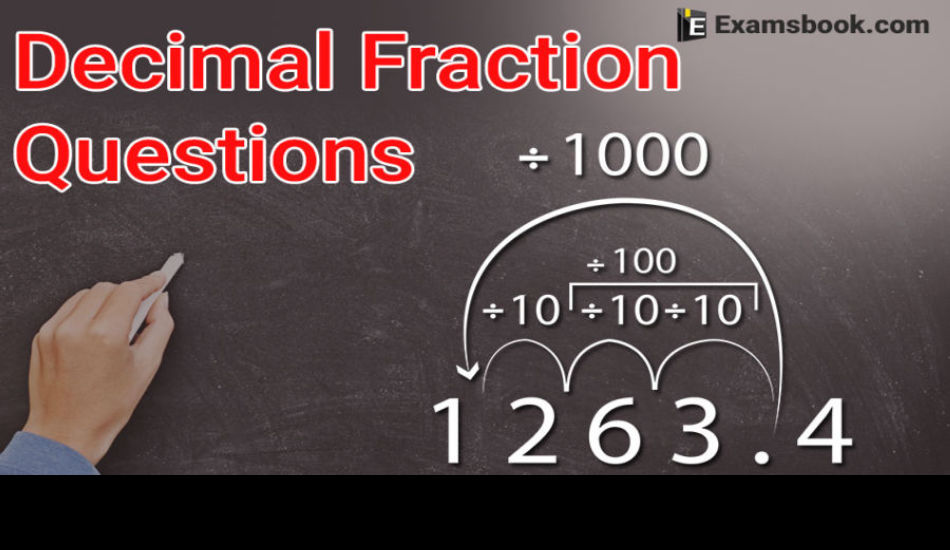Decimal fraction questions have been asked in many competitive exams and there are strong chances to ask decimal fraction questions aptitude questions again in SSC, Bank and other competitive exams. Students should prepare for exams by practicing decimal fraction questions and answers.

You should know the complete detail of decimal fraction with its types.

Decimal fraction problems are important and 1-2 questions asked in exams every year, try to solve decimal fraction aptitude questions and answers for better results in upcoming competitive exams.

You can learn to use decimal fraction formula with examples to improve your performance as well.

## Important Decimal Fraction Aptitude Questions and Answers:

1. When .36 is written in simplest fractions form, the sum of the numerator and the denominator is:

(A) 15

(B) 30

(C) 45

(D) 60

Ans .   A

2. Which of the following fractions is the smallest?

(A)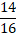(B)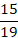(C)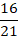(D)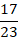Ans .   B

3. Which of the following fractions is less than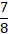and greater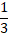?

(A)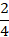(B)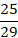(C)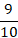(D)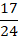Ans .   D

4. 337.62+8.591+34.4 =?

(A) 371.722

(B) 391.622

(C) 380.611

(D) 463.94

Ans .   C

5. 34.95+240.016+23.98=?

(A) 298.1057

(B) 298.222

(C) 298.946

(D) 299.09

Ans .   C

6. 48.95 - 32.006 =?

(A) 16.091

(B) 16.34

(C) 16.97

(D) 16.944

Ans .   D

7. 12.1212 + 17.0005 – 9.1102 =?

(A) 20.0017

(B) 20.0216

(C) 20.0115

(D) 20.1115

Ans .   C

8. 3889 + 12.952 –? = 3854.002

(A) 47.015

(B) 47.641

(C) 47.943

(D) 47.95

Ans .   D

Ask me in the comment section, if you face any problem while solving Decimal fraction questions. Visit next page for more decimal fraction questions and answers.